### PHENOMENA OF SLOW VARIATION

WALTER RITZ

#### Annales 223 (Oeuvres 382)

We will transform the expression of F starting from the hypothesis that the developments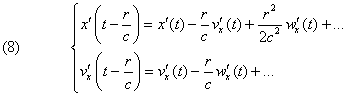(Oeuvres 383) are very convergent and we want to approximately calculate F up to terms of third order (in c-3).

Let’s denote by ρ the vector whose components are x(t)-x'(t), y(t)-y'(t), z(t)-z'(t) , in other words the present distance between two electrical points, and let Aρ be the component of the vector A in the direction ρ. We will have then, according to (2),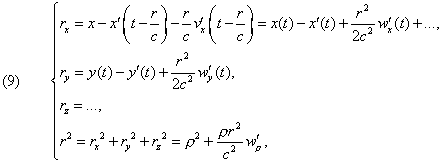from which finally(11)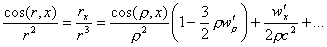Likewise

(12)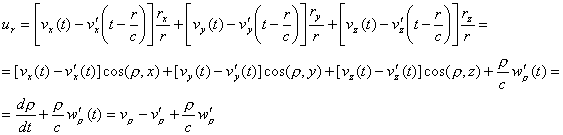The expressions u2, ur2 entering F only with the factor 1/c2, their expansion will stop with the first term: u2 will be the square of the current relative speed of

the two points, that is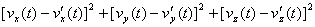and(Oeuvres 384) Neglecting the terms which in the expression (VI) for F would be multiplied by 1/c3, it finally comes to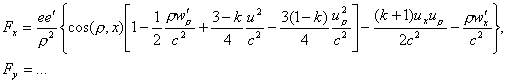(13)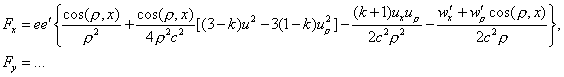where, let’s say it again, all the magnitudes should be taken at the moment t. We thus found again the very convenient form of action at a distance. We can see that the action is not equal to the reaction, but the difference is a term containing only the accelerations and not the speeds.

This formula can be writtenNow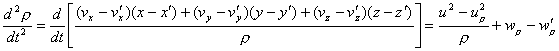andin the manner that the first parenthesis is written , since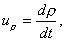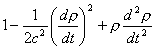[ = 0?  trans. Ed.]

[Need something like “= 0” for this expression to make sense. Comments welcome]

(Oeuvres 385) as in the well known law of Weber; the second represents Riemann’s law (), the third is equal toOn the whole, concerning the speeds, we are now in the presence of the most general linear combination of the two laws proposed by Weber and Riemann for the explanation of electrical phenomena. Yet, we know, and it is easy to check, that these two formulas can be written in the classical form,

where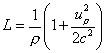for Weber, and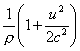for Riemann.

Their linear combination will susceptibly be of the same form, and, in putting

(14)we will have

(15)Formulating very special hypotheses about the nature of the current of conduction, and which today should be considered as inadmissible, at least for the electrolytes, Weber and Riemann have shown that their formulas represent well known laws of electrodynamics and of induction in closed circuits. We will demonstrate that this result is independent of these special hypotheses () and it is extended even to actions of closed currents of the free ions of cathode rays for example, and to actions (Oeuvres 386) that static electricity in motion exerts on a magnet (in other words, a system of closed currents). In all the phenomena of this kind observed until now, there is at least one closed circuit and this is a very important point. As for phenomena of induction in open circuits, very fast, they depend uniquely on the term containing the accelerations w'; yet we will see that this term is the same in both theories: it is entirely determined by the experiments made on the hertzian oscillations, and we can say that all experimental confirmations of Maxwell’s and Lorentz’s theory are based only on this term and on the fact of propagation. As for the manner that these speeds enter the formula of the elementary force,

It is interesting to compare this formula to that resulting from Lorentz’s theory when we develop it up to the terms of 2nd order. It comes, according to (III)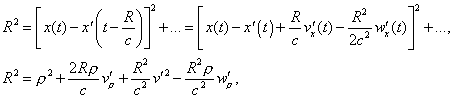the quantities v', w' being taken for the time t, we resolve it in relation to R and re-expand it; it will then becomeLikewise, to the terms of the order 1/c2 [because,appearing in (IV) with the factor 1/c, this approximation is sufficient],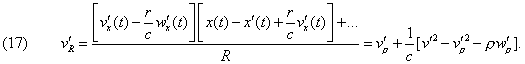(Oeuvres 387) The expression of potentials Φ, A given by Messrs. Liénard and Wiechert () become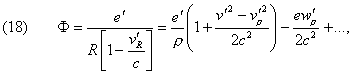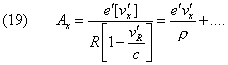The component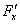of the force, of which the general expression for the potentials is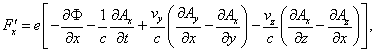becomes, to the terms of the order 1/c2,We see that this expression agrees with (13) only in what concerns the electrostatic term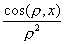and the accelerations. We can put it in the form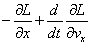as Mr. Schwarzschild demonstrated, by setting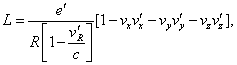(Oeuvres 388) that isB. Riemann, Schwere; Elektrizität und Magnetismus, Hannover, 1876, paragraphs 98, 99. On this subject see Enzyklopaedie der Mathematische Wissenschaftem, vol. V, Art. 12, p. 38 and 47 (Reiff and Sommerfeld).

 It is what different scientists remarked, at least concerning particular cases. See Poincaré: Electricité et Optique, p. 263 (2nd ed.)

 Liénard, L’Eclairage électrique (Electric lighting), vol. XVI, 1898, pp. 5, 53, and 106. Wiechert, Archives néerlandaises (Netherlands Archives), vol. V, 1990, p. 549. For the case when the electricity is distributed in a homogenous manner and fixed to solid in translation, we can use these same formulas, no matter what the distance is, by replacing e, e' by de, de'; it is what we will see by developing directly under the sum sign in the general formula of potentials (formulas (XII) and (XIII) in the 1st part).